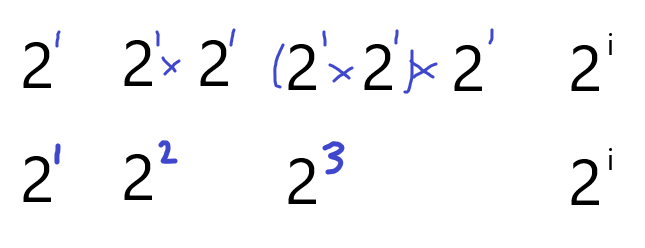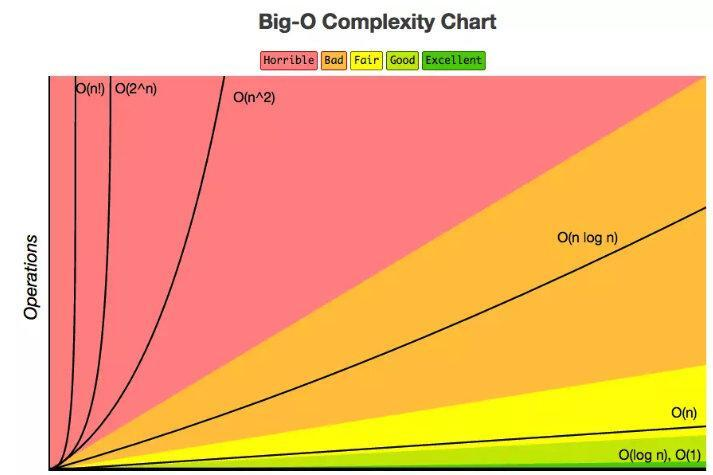# 算法的时间与空间复杂度## 事前分析法

### 大 O 时间复杂度

#### 几个原则

2(n^2) =n^2

test(n) {  //时间复杂度n^3 for(int i = 0; i < n ; i++){   for(int i = 0; i < n ; i++){     for(int i = 0; i < n ; i++){            print(n);     }   } } //时间复杂度n^2 for(int i = 0; i < n ; i++){   for(int i = 0; i < n ; i++){     print(n);   } } //时间复杂度n for(int i = 0; i < n ; i++){   print(n); }}

#### 常见复杂度

o(1)

i = i + 1;

o(n)

test(n){  for(int i = 0 ;i < n;i++){    print(i);  }}

o(n^2)

test(n){  for(int i = 0 ;i < n;i++){    print(i);    for(int j = 0 ;j < n;j++){      print(i);    }  }}

o(log2n)

PS:如果 ax =N（a>0，且 a≠1），那么数 x 叫做以 a 为底 N 的对数，记作 x=logaN，读作以 a 为底 N 的，其中 a 叫做对数的底数，N 叫做真数。

test(n) {  int i = 1;  while (i <= n) {    i = 2 * i;  }}## 空间复杂度

test(n) {  //在内存中开辟了一个长度为n的数组  List array  =  List(n);  print(array.length);}## 评论